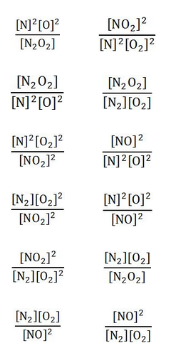# Problem: Match each chemical equation below with the correct equilibrium constant expression.Chemical Equation                                           Equilibrium Constant Expression2NO(g) ⇌ N2(g) + O2(g)                                     K =       N2(g) + O2(g) ⇌ N2O2(g)                                   K =        2NO2(g) ⇌ N2(g) + 2O2(g)                                 K =N2(g) + 2O2(g) ⇌ 2NO2(g)                                 K =N2(g) + O2(g) ⇌ 2NO2(g)                                   K =N2O2(g) ⇌ N2(g) + O2(g)                                    K =

###### FREE Expert Solution

Equilibrium Expression:

Note:

• Solids and liquids are ignored in the expression.
• Coefficients in the balanced reaction become superscripts.

Chemical Equations

90% (460 ratings)###### Problem Details

Match each chemical equation below with the correct equilibrium constant expression.

Chemical Equation                                           Equilibrium Constant Expression

2NO(g) ⇌ N2(g) + O2(g)                                     K =

N2(g) + O2(g) ⇌ N2O2(g)                                   K =

2NO2(g) ⇌ N2(g) + 2O2(g)                                 K =

N2(g) + 2O2(g) ⇌ 2NO2(g)                                 K =

N2(g) + O2(g) ⇌ 2NO2(g)                                   K =

N2O2(g) ⇌ N2(g) + O2(g)                                    K =